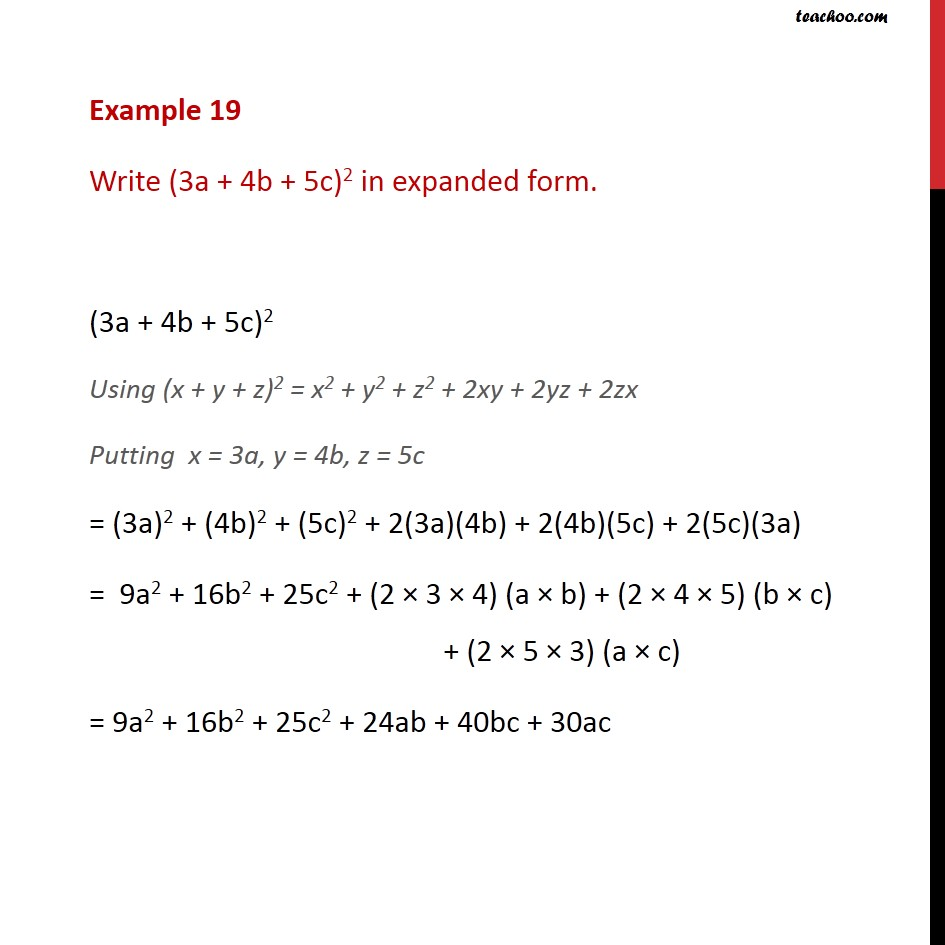Examples

Chapter 2 Class 9 Polynomials
Serial order wiseGet live Maths 1-on-1 Classs - Class 6 to 12

### Transcript

Example 19 Write (3a + 4b + 5c)2 in expanded form. (3a + 4b + 5c)2 Using (x + y + z)2 = x2 + y2 + z2 + 2xy + 2yz + 2zx Putting x = 3a, y = 4b, z = 5c = (3a)2 + (4b)2 + (5c)2 + 2(3a)(4b) + 2(4b)(5c) + 2(5c)(3a) = 9a2 + 16b2 + 25c2 + (2 × 3 × 4) (a × b) + (2 × 4 × 5) (b × c) + (2 × 5 × 3) (a × c) = 9a2 + 16b2 + 25c2 + 24ab + 40bc + 30ac